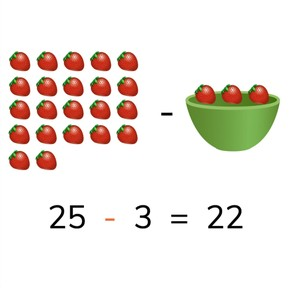Subtraction to 30 without regrouping

# Subtraction to 30 without regrouping8,000 schools use Gynzy92,000 teachers use Gynzy1,600,000 students use Gynzy

## General

Students learn to subtract numbers to 30 without regrouping tens.

2.OA.A.1

## Relevance

It is important for students to learn how to solve subtraction problems to 30, to determine how many they have left.

## Introduction

Practice the number line to 30 by asking students to determine which balloon fits the question mark on the number line. Then practice another subtraction problem on the number line to 20.

## Development

First discuss the importance of being able to subtract numbers to 30. The learning goal is then presented in three ways, visually, in the abstract, and in story form. You can select the method which best applies to your classroom situation by using the blue menu in the bottom right of the page. Otherwise, you can start at the first page with the visual support. Using the strawberries shown on the interactive whiteboard, discuss how to solve a subtraction problem. Next ask students to practice a subtraction problem with visual support. Then the abstract subtraction problem is given. Tell students that they can solve these by counting back in their heads, or by imagining a number line, or even by using blocks or a rekenrek. Have students practice with a set of abstract subtraction problems. Then the steps of solving a story problem are given, discuss these step by step and solve the given story problem together. Then ask students to work in pairs and determine the difference in the next story problem. Discuss their answers as a class.

Check that students are able to subtract numbers to 30 by asking the following questions:
- Why is it useful to be able to subtract numbers to 30?
- How do you determine which number is the first number in a subtraction problem?

## Guided practice

Students are first given a subtraction problem with visual support, then one without visual support, and are then given a story problem to solve.

## Closing

As a class, do one of each type of subtraction problem (visual, abstract and story). Discuss the different strategies you use for each kind of problem.

## Teaching tips

Students who have difficulty can be supported by using blocks or other manipulatives, or by writing out a number line and using that to count on.

## Instruction materials

Optional- blocks or other manipulatives

### The online teaching platform for interactive whiteboards and displays in schools

• Save time building lessons

• Manage the classroom more efficiently

• Increase student engagement